# Related Videos

## Simplify the expression $\frac{x^2+x-2}{x^2+5x+6}$

Go!
1
2
3
4
5
6
7
8
9
0
a
b
c
d
f
g
m
n
u
v
w
x
y
z
.
(◻)
+
-
×
◻/◻
/
÷
2

e
π
ln
log
log
lim
d/dx
Dx
|◻|
=
>
<
>=
<=
sin
cos
tan
cot
sec
csc

asin
acos
atan
acot
asec
acsc

sinh
cosh
tanh
coth
sech
csch

asinh
acosh
atanh
acoth
asech
acsch

### Videos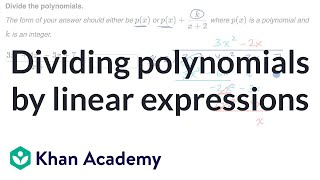### Dividing polynomials by linear expressions | Algebra 2 | Khan Academy

https://www.youtube.com/watch?v=G2VBRwXq1q4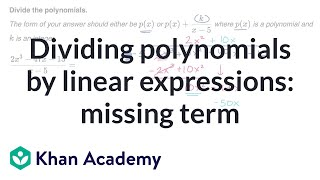### Dividing polynomials by linear expressions: missing term | Algebra 2 | Khan Academy

https://www.youtube.com/watch?v=QTZ5Dn0t6M4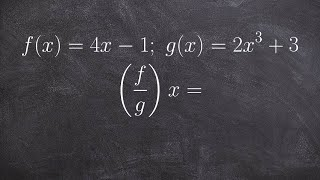### Algebra 2 - Find the domain from division of two functions, f(x)=4x -1; g(x)=2x^3 +3; (f/g)(x)=?

https://www.youtube.com/watch?v=g53Tpfx3v7E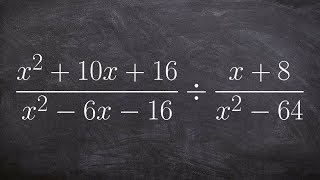### Algebra 2 - Divide two rational expressions by simplifying (x^2+10x+16/x^2‐6x‐16)/(x+8/x^2‐64)

https://www.youtube.com/watch?v=o5jolD6rPco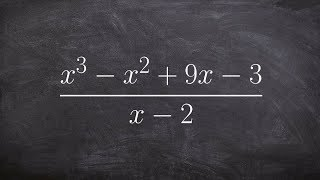### Algebra 2-using synthetic division to divide two polynomials with a remainder (x^3‐x^2+9x‐3)/(x‐2)

https://www.youtube.com/watch?v=qzTkg7iFAbU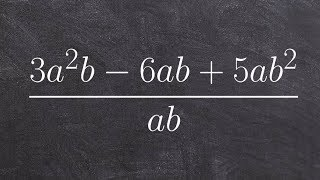### Dividing a polynomial by a monomial

https://www.youtube.com/watch?v=D4aowg1urlQ
$\frac{x^2+x-2}{x^2+5x+6}$

### Main topic:

Polynomial long division

~ 0.05 s